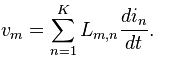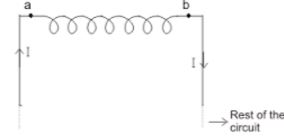# Inductance

Inductance is typified by the behavior of a coil of wire in resisting any change of electric current through the coil.

If a changing flux is linked with a coil of a conductor there would be an emf induced in it. The property of the coil of inducing emf due to the changing flux linked with it is known as inductance of the coil. Due to this property all electrical coil can be referred as inductor. In other way, an inductor can be defined as an energy storage device which stores energy in form of magnetic field.

An electronic component that is intended to add inductance to a circuit is called an inductor. Inductors are typically manufactured from coils of wire. This design delivers two desired properties, a concentration of the magnetic field into a small physical space and a linking of the magnetic field into the circuit multiple times.The relationship between the self-inductance L of an electrical circuit, the voltage v(t), and the current i(t) through the circuit is

V(t) = L(di/dt)

The generalization to the case of K electrical circuits with currents im and voltages vm reads